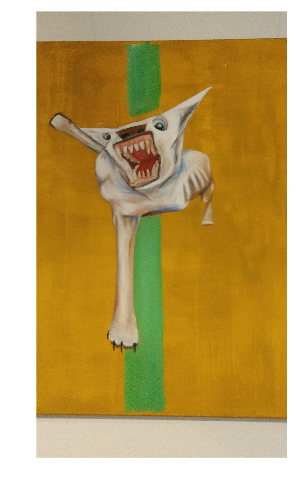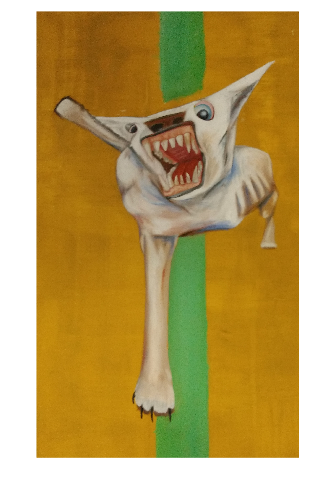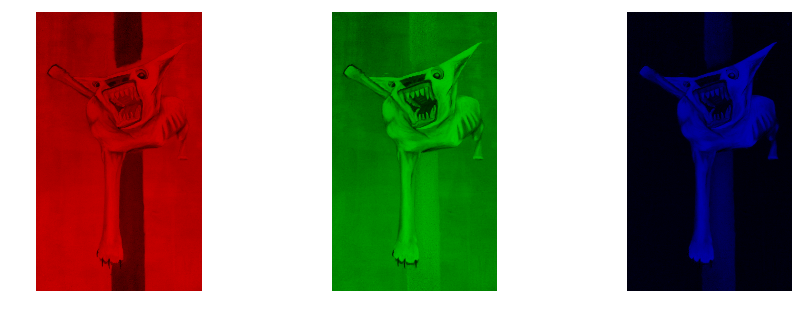# Image Processing with Numpy

Published: 23/10/2016

I recently had to computationally alter some images, an ended up getting interested in some of the basic image manipulation techniques. The result is this post.

In python, there are a number of powerful libraries that make image processing easy, such as OpenCV, SciKit-Image and Pillow. For anyone thinking about doing serious image processing, they should be the first place to look.

However, I am not planning on putting anything into production. Instead the goal of this post is to try and understand the fundamentals of a few simple image processing techniques. Because of this, I am going to stick to using numpy to preform most of the manipulations, although I will use other libraries now and then.

In :
import numpy as np
import matplotlib.pylab as plt

%matplotlib inline


In :
im = plt.imread("BTD.jpg")

im.shape

Out:
(4608, 2592, 3)

We see that image is loaded into an array of dimension 4608 x 2592 x 3.

The first two indices represent the Y and X position of a pixel, and the third represents the RGB colour value of the pixel. Let's take a look at what the image is of

In :
def plti(im, h=8, **kwargs):
"""
Helper function to plot an image.
"""
y = im.shape
x = im.shape
w = (y/x) * h
plt.figure(figsize=(w,h))
plt.imshow(im, interpolation="none", **kwargs)
plt.axis('off')

plti(im)It is a photo of a painting of a dog. The painting itself was found in a flat in London I lived in may years ago, abandoned by its previous owner. I don't know what the story behind it is. If you do, please get in touch.

We can see that whichever bumbling fool took that photo of the painting also captured a lot of the wall. We can crop the photo so we are only focused on the painting itself. In numpy, this is just a matter of slicing the image array

In :
im = im[400:3800,:2000,:]
plti(im)# Colours¶

Each pixel of the image is represented by three integers: the RGB value of its colour. Splitting the image into seperate colour components is just a matter of pulling out the correct slice of the image array:

In :
fig, axs = plt.subplots(nrows=1, ncols=3, figsize=(15,5))

for c, ax in zip(range(3), axs):
tmp_im = np.zeros(im.shape, dtype="uint8")
tmp_im[:,:,c] = im[:,:,c]
ax.imshow(tmp_im)
ax.set_axis_off()When using matplotlib's imshow to display images, it is important to keep track of which data type you are using, as the colour mapping used is data type dependent: if a float is used, the values are mapped to the range 0-1, so we need to cast to type "uint8" to get the expected behavior. A good discussion of this issue can be found here here.

In my first edition of this post I made this mistake. Thanks to commenter Rusty Chris Holleman for pointing out the problem.

## Colour Transformations¶

Representing colour in this way, we can think of each pixel as a point in a three dimensional space. Thinking of colour this way, we can apply various transformations to the colour "point". An interesting example of these is "rotating" the colour.

There is some subtleties - a legal colours officially exist as integer points in a three dimensional cube of side lengths 255. It is possible that a rotation could push a point out of this cube. To get around this I apply a sigmoid transformation to the data, a mapping from the range 0-1 to the full real line. Having applied this transformation we apply the rotation matrix then transform back to colour space.

The rotation matrix is applied pixel-wise to to the image using numpy's Einstein notation function, which I hadn't used before but, but make the operation concise. It is explained well in this post.

The following functions apply a sigmoid to the images colour space, and rotate it about the red axis by some angle, before returning the image to normal colour space.

In :
def do_normalise(im):
return -np.log(1/((1 + im)/257) - 1)

def undo_normalise(im):
return (1 + 1/(np.exp(-im) + 1) * 257).astype("uint8")

def rotation_matrix(theta):
"""
3D rotation matrix around the X-axis by angle theta
"""
return np.c_[
[1,0,0],
[0,np.cos(theta),-np.sin(theta)],
[0,np.sin(theta),np.cos(theta)]
]

im_normed = do_normalise(im)
im_rotated = np.einsum("ijk,lk->ijl", im_normed, rotation_matrix(np.pi))
im2 = undo_normalise(im_rotated)

plti(im2)